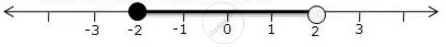Share

# For the Given Inequations Graph the Solution Set on the Real Number Line X - 1 < 3 - X <= 5 - Mathematics

Course

#### Question

For the given inequations graph the solution set on the real number line

x - 1 < 3 -  x <= 5

#### Solution

x - 1 < 3 -  x <= 5

x - 1 < 3 - x  and 3 - x <= 5

2x < 4  and -x <= 2

x < 2  and x >= -2

The solution set on the real number line isIs there an error in this question or solution?